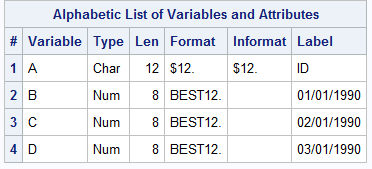## Import data from Excel where variable name is date

Hi,

I want to import an excel files to SAS (v9.4).  The excel is formatted as such: the 1st column being people' names (titled Name) and the rest of the columns being "1/1/1990    2/1/1990   3/1/1990........".  The data is each person's sales in each month.  The folloiwng code was used:

PROC IMPORT OUT= file_SAS DATAFILE= "directory\file_excel.xls"
DBMS=xls REPLACE
SHEET='Sheet1';
GETNAMES=YES;
namerow=8; /*the variable names on row 8*/
datarow=9;
RUN;

After imported, file_SAS only shows the "Name" as the variable name in the 1st column.  The remaining date variables became alphabet C, D, E, .....  Is there any way for SAS to keep the variable name without using rename (I have hundres of months)?  Or any suggestion about importing this kind of data structure?  Any suggestions welcomed!  Thank you.

1 ACCEPTED SOLUTION

Accepted Solutions

## Re: Import data from Excel where variable name is date

Read the data using GETNAMES=no. Then read the names.  You can then use names you read to generate new variable names, labels or whatever you want.

``````proc import
out=want replace
datafile= "&path\excel_file.xls"  dbms=xls
;
sheet='sheet1';
getnames=no;
datarow=9;
run;

proc import
out=names replace
datafile= "&path\excel_file.xls"   dbms=xls
;
sheet='sheet1';
getnames=no;
datarow=8;
run;

proc transpose data=names(obs=1) out=labels;
var _all_;
run;

proc sql noprint ;
select catx('=',_name_,catq('1as',col1))
into :labels separated by ' '
from labels
;
quit;

proc datasets nolist lib=work;
modify want;
label &labels;
run;

proc contents data=want; run;

``````5 REPLIES 5

## Re: Import data from Excel where variable name is date

Those wouldn't be valid SAS variable names, but I'd expect them to be assigned as the labels. Did that happen?

Run a proc contents if needed to see the labels.

I highly recommend against this data structure, its known as storing data in variable names and not considered tidy data. You should normalize it, or make it long once in SAS to work with it.

If you really, really want, you can try setting the validvarname option before the import to see how SAS reads the variable name.

``options validvarname=ANY;``

If it does read it correctly as a date then it you'll have to refer to your variables as '1/1/1990'n when programming.

## Re: Import data from Excel where variable name is date

The excel is from other source so I don't get to decide the data structure. I don't have to use the data structure, just want to import the dataset while keeping the date information. SAS did not assign those as labels. Both the names and labels were C, D, E,... And I tried validvarname=ANY option and it did not work.

## Re: Import data from Excel where variable name is date

That's too bad. The unfortunate thing in dealing with Excel and Proc Import is you have very little control over the data import.

The general workaround is to save the data to CSV, use proc import, get the code from the log and customize that.

Another possible option is if you know the name structure, ie 1/1/1990 and it increases by year or date you can create customizable names/labels after the fact but that's as bit risky if there's any chance the names are swapped or one is missing.

You can copy the variable names, paste special and transpose them and then use that as a source to label the variables. In fact I'd use Excel to create the code using the concatenate function.

## Re: Import data from Excel where variable name is date

Read the data using GETNAMES=no. Then read the names.  You can then use names you read to generate new variable names, labels or whatever you want.

``````proc import
out=want replace
datafile= "&path\excel_file.xls"  dbms=xls
;
sheet='sheet1';
getnames=no;
datarow=9;
run;

proc import
out=names replace
datafile= "&path\excel_file.xls"   dbms=xls
;
sheet='sheet1';
getnames=no;
datarow=8;
run;

proc transpose data=names(obs=1) out=labels;
var _all_;
run;

proc sql noprint ;
select catx('=',_name_,catq('1as',col1))
into :labels separated by ' '
from labels
;
quit;

proc datasets nolist lib=work;
modify want;
label &labels;
run;

proc contents data=want; run;

``````## Re: Import data from Excel where variable name is date

Thanks you guys for your help!

Discussion stats
• 5 replies
• 2353 views
• 2 likes
• 3 in conversation